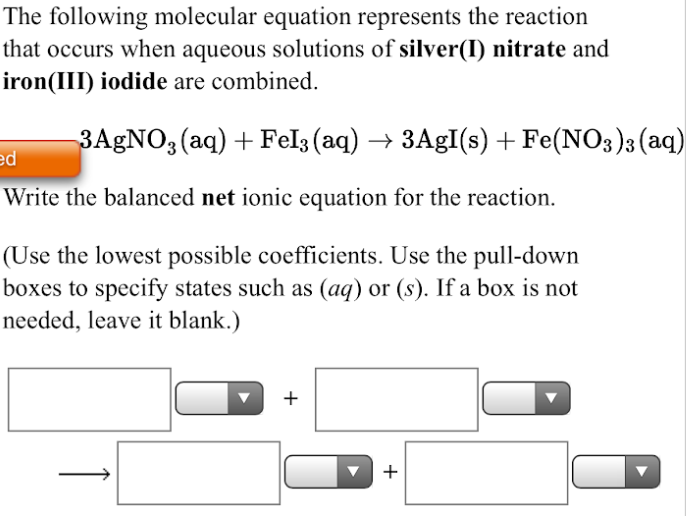# The following molecular equation represents the reaction that occurs when aqueous solutions of silver(I) nitrate and iron(III) iodide are combined. 3AgNO3(aq) + FeI3(aq) → 3AgI(s) + Fe(NO3)3(aq) Write the balanced net ionic equation for the reaction. (Use the lowest possible coefficients. Use the pull-down boxes to specify states such as (aq) or (s) . If a box is not needed, leave it blank.)